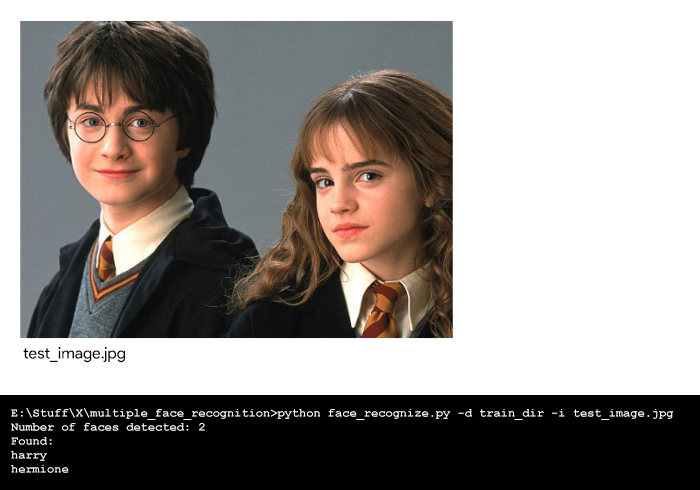# Python | Multiple Face Recognition using dlib

This article aims to quickly build a Python face recognition program to easily train multiple images per person and get started with recognizing known faces in an image.

In this article, the code uses ageitgey’s face_recognition API for Python. This API is built using dlib’s face recognition algorithms and it allows the user to easily implement face detection, face recognition and even real-time face tracking in your projects or from the command line.

Library Installation:
At first, make sure the required libraries are installed on your computer.

`pip install face_recognition Scikit-learn dlib docopt`

If you want dlib to use CUDA on GPU, make sure CUDA and cuDNN are installed correctly then install dlib using pip. For detailed instructions for installation on different platforms, check out face_recognition’s Installation Guide.

Now, create a directory with the training images for each person in the below format. Make sure that you’ve enough training images (at least 5 for each person) otherwise Support Vector Classifier (SVC) (that is used in this program – produces meaningless results on very small datasets.

File structure:

```face_recognize.py
test_image.jpg
train_dir/
person_1/
person_1_face-1.jpg
person_1_face-2.jpg
.
.
person_1_face-n.jpg
person_2/
person_2_face-1.jpg
person_2_face-2.jpg
.
.
person_2_face-n.jpg
.
.
person_n/
person_n_face-1.jpg
person_n_face-2.jpg
.
.
person_n_face-n.jpg
```

Face Encoding:
The face_recognition API generates face encodings for the face found in the images. A face encoding is basically a way to represent the face using a set of 128 computer-generated measurements. Two different pictures of the same person would have similar encoding and two different people would have totally different encoding.

After all the face encodings are generated, Support Vector Classifier (SVC) with scikit-learn is trained on the face encodings along with their labels from all the known faces in the training directory. Finally, the API detects all the faces in the test image you provide and the trained SVC predicts all the known faces in the test image.

Code : To recognize multiple faces.

 `""" ` `Usage: ` `  ``face_recognize.py -d -i ` ` `  `Options: ` `  ``-h, --help                     Show this help ` `  ``-d, --train_dir =   Directory with  ` `                                 ``images for training ` `  ``-i, --test_image = Test image ` `"""` ` `  `# importing libraries ` `import` `face_recognition ` `import` `docopt ` `from` `sklearn ``import` `svm ` `import` `os ` ` `  `def` `face_recognize(``dir``, test): ` `    ``# Training the SVC classifier ` `    ``# The training data would be all the  ` `    ``# face encodings from all the known  ` `    ``# images and the labels are their names ` `    ``encodings ``=` `[] ` `    ``names ``=` `[] ` ` `  `    ``# Training directory ` `    ``if` `dir``[``-``1``]!``=``'/'``: ` `        ``dir` `+``=` `'/'` `    ``train_dir ``=` `os.listdir(``dir``) ` ` `  `    ``# Loop through each person in the training directory ` `    ``for` `person ``in` `train_dir: ` `        ``pix ``=` `os.listdir(``dir` `+` `person) ` ` `  `        ``# Loop through each training image for the current person ` `        ``for` `person_img ``in` `pix: ` `            ``# Get the face encodings for the face in each image file ` `            ``face ``=` `face_recognition.load_image_file( ` `                ``dir` `+` `person ``+` `"/"` `+` `person_img) ` `            ``face_bounding_boxes ``=` `face_recognition.face_locations(face) ` ` `  `            ``# If training image contains exactly one face ` `            ``if` `len``(face_bounding_boxes) ``=``=` `1``: ` `                ``face_enc ``=` `face_recognition.face_encodings(face)[``0``] ` `                ``# Add face encoding for current image  ` `                ``# with corresponding label (name) to the training data ` `                ``encodings.append(face_enc) ` `                ``names.append(person) ` `            ``else``: ` `                ``print``(person ``+` `"/"` `+` `person_img ``+` `" can't be used for training"``) ` ` `  `    ``# Create and train the SVC classifier ` `    ``clf ``=` `svm.SVC(gamma ``=``'scale'``) ` `    ``clf.fit(encodings, names) ` ` `  `    ``# Load the test image with unknown faces into a numpy array ` `    ``test_image ``=` `face_recognition.load_image_file(test) ` ` `  `    ``# Find all the faces in the test image using the default HOG-based model ` `    ``face_locations ``=` `face_recognition.face_locations(test_image) ` `    ``no ``=` `len``(face_locations) ` `    ``print``(``"Number of faces detected: "``, no) ` ` `  `    ``# Predict all the faces in the test image using the trained classifier ` `    ``print``(``"Found:"``) ` `    ``for` `i ``in` `range``(no): ` `        ``test_image_enc ``=` `face_recognition.face_encodings(test_image)[i] ` `        ``name ``=` `clf.predict([test_image_enc]) ` `        ``print``(``*``name) ` ` `  `def` `main(): ` `    ``args ``=` `docopt.docopt(__doc__) ` `    ``train_dir ``=` `args[``"--train_dir"``] ` `    ``test_image ``=` `args[``"--test_image"``] ` `    ``face_recognize(train_dir, test_image) ` ` `  `if` `__name__``=``=``"__main__"``: ` `    ``main() `

How to run it on the terminal:

```python face_recognize.py -d train_dir -i test_image.jpg
```

Output:My Personal Notes arrow_drop_upCheck out this Author's contributed articles.

If you like GeeksforGeeks and would like to contribute, you can also write an article using contribute.geeksforgeeks.org or mail your article to contribute@geeksforgeeks.org. See your article appearing on the GeeksforGeeks main page and help other Geeks.

Please Improve this article if you find anything incorrect by clicking on the "Improve Article" button below.